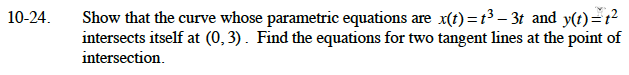### Home > CALC > Chapter 10 > Lesson 10.1.3 > Problem10-24

10-24.Notice that y(t) = t2. At the point (0, 3), t2 = 3. How many solutions does this equation have?
What is the value of x(t) for these solutions?

To compute the slopes of the tangent lines at this point, use: